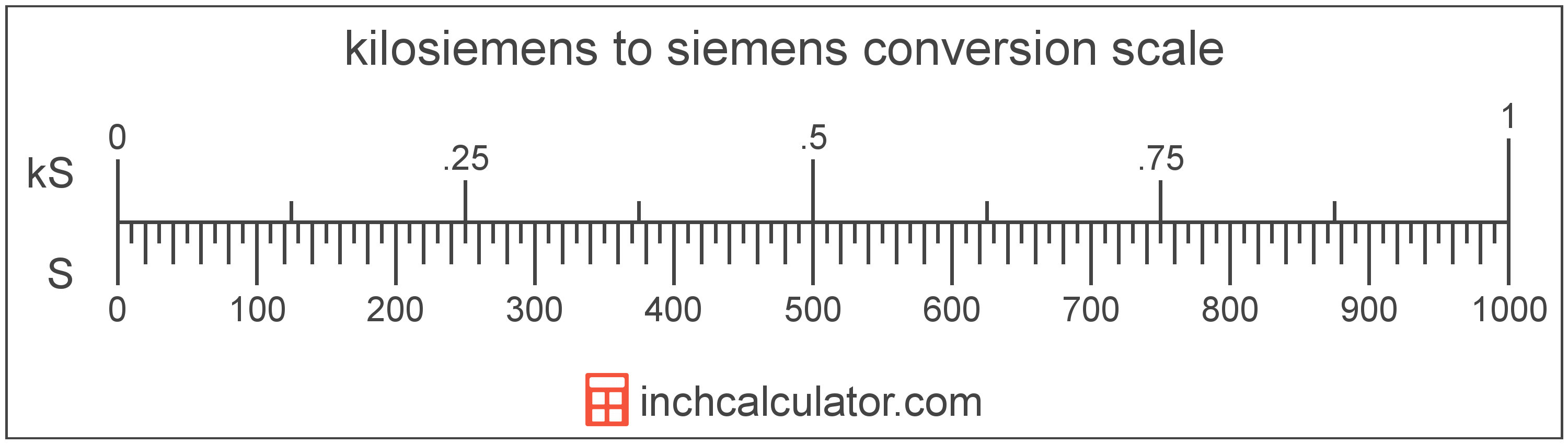# Siemens to Kilosiemens Conversion

Enter the electrical conductance in siemens below to get the value converted to kilosiemens.

Results in Kilosiemens:1 S = 0.001 kS

## How to Convert Siemens to KilosiemensTo convert a siemens measurement to a kilosiemens measurement, divide the electrical conductance by the conversion ratio.

Since one kilosiemens is equal to 1,000 siemens, you can use this simple formula to convert:

kilosiemens = siemens ÷ 1,000

The electrical conductance in kilosiemens is equal to the siemens divided by 1,000.

For example, here's how to convert 5,000 siemens to kilosiemens using the formula above.
5,000 S = (5,000 ÷ 1,000) = 5 kS

## Siemens

One siemens is the electrical conductance equal to an increase in current of one ampere for every increase in electrical potential difference of one volt. The siemens is the SI defined unit equal to the mho, and is the reciprocal of the resistance in ohms.

The siemens is the SI derived unit for electrical conductance in the metric system. Siemens can be abbreviated as S; for example, 1 siemens can be written as 1 S.

## Kilosiemens

The kilosiemens is the SI defined unit equal to the kilomho. One kilosiemens is the electrical conductance equal to 1,000 siemens, which are equal to one ampere per volt.

The kilosiemens is a multiple of the siemens, which is the SI derived unit for electrical conductance. In the metric system, "kilo" is the prefix for 103. Kilosiemens can be abbreviated as kS; for example, 1 kilosiemens can be written as 1 kS.

## Siemens to Kilosiemens Conversion Table

Siemens measurements converted to kilosiemens
Siemens Kilosiemens
1 S 0.001 kS
2 S 0.002 kS
3 S 0.003 kS
4 S 0.004 kS
5 S 0.005 kS
6 S 0.006 kS
7 S 0.007 kS
8 S 0.008 kS
9 S 0.009 kS
10 S 0.01 kS
20 S 0.02 kS
30 S 0.03 kS
40 S 0.04 kS
50 S 0.05 kS
60 S 0.06 kS
70 S 0.07 kS
80 S 0.08 kS
90 S 0.09 kS
100 S 0.1 kS
200 S 0.2 kS
300 S 0.3 kS
400 S 0.4 kS
500 S 0.5 kS
600 S 0.6 kS
700 S 0.7 kS
800 S 0.8 kS
900 S 0.9 kS
1,000 S 1 kS